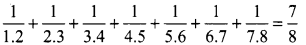Students can Download Maths Chapter 1 Rational Numbers Intext Questions and Answers, Notes Pdf, Samacheer Kalvi 8th Maths Book Solutions Guide Pdf helps you to revise the complete Tamilnadu State Board New Syllabus and score more marks in your examinations.## Tamilnadu Samacheer Kalvi 8th Maths Solutions Term 1 Chapter 1 Rational Numbers Intext Questions

Exercise 1.1
Try This (Textbook Page No. 6)
Question 1.
Is the number $$\frac{7}{-5}$$ a fraction or a rational number ? Why?
Solution:
$$\frac{7}{-5}$$ is a rational number
Because of rational number is a rational number which is of the form $$\frac{p}{q}$$ , q ≠ 0 and p and q are integers.
But fraction is part of a whole.

Question 2.
Write any 6 rational numbers of your choice.
Solution:
0, $$\frac{-1}{2}$$, $$\frac{1}{2}$$, $$\frac{3}{4}$$, $$\frac{6}{7}$$, -5, 6

Try This (Textbook Page No. 7)

Question 1.
Explain why the following statements are true?
(i) 0.8 = $$\frac{4}{5}$$
(ii) 1.4 > $$\frac{1}{4}$$
(iii) 0.74 < $$\frac{3}{4}$$ (iv) 0.4 > 0.386
(v) 0.096 < 0.24
(vi) 1.128 = 0.1280
Solution: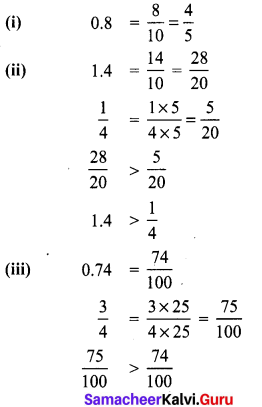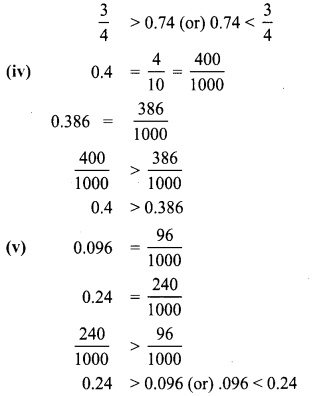(vi) 0.128 = $$\frac{128}{1000}=\frac{1280}{10000}$$
0.1280 = $$\frac{1280}{10000}$$
∴ Both are equal. i.e., 0.128 = 0.1280Try This (Textbook Page No. 9)

Question 1.
Which of the pairs are equivalent rational numbers?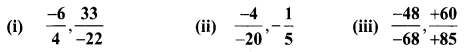Solution: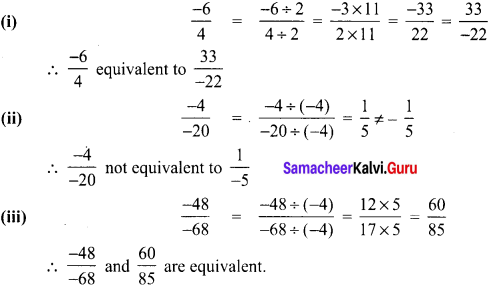Question 2.
Find the standard form of
(i) $$\frac{36}{-96}$$
(ii) $$\frac{-56}{-72}$$
(iii) $$\frac{27}{18}$$
Solution: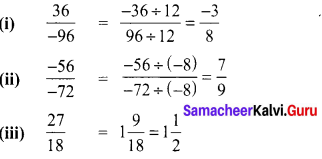Question 3.
Mark the following rational numbers on a number line.
(i) $$\frac{-2}{3}$$
(ii) $$\frac{-8}{-5}$$
(iii) $$\frac{5}{-4}$$
Solution:
(i) $$\frac{-2}{3}$$ lies between 0 and -1.
The unit part between 0 and -1 is divided into 3 equal parts and second part is taken.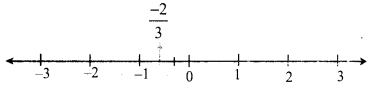(ii) $$\frac{-8}{-5}=1 \frac{3}{5}$$
$$1 \frac{3}{5}$$ lies between 1 and 2. The unit part between 1 and 2 is divided into 5 equal parts and the third part is taken.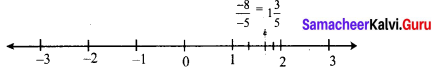(iii) $$\frac{5}{-4}=-\frac{5}{4}=-1 \frac{1}{4}$$
$$-1 \frac{1}{4}$$ lies between -1 and The unit part between -1 and -2 is divided into four equal parts and the first part is taken.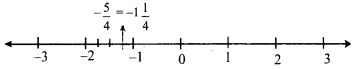Try This (Textbook Page No. 15)

Question 1.
Are there any rational numbers between $$\frac{-7}{11}$$ and $$\frac{6}{-11}$$ ?
Solution: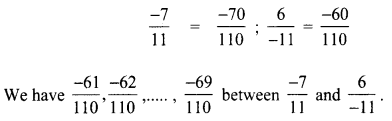Try This (Textbook Page No. 19)

Question 1.
Divide: (i) 5 by $$\frac{-7}{3}$$
(ii) $$\frac{-7}{3}$$ by 5
Solution: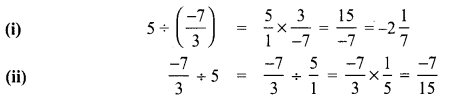Exercise 1.2
Try This (Textbook Page No. 25)

Question 1.
The closure property on integers holds for subtraction and not for division. What about rational numbers? Verify.
Solution:
Let 0 and $$\frac{1}{2}$$ be two rational numbers 0 – $$\frac{1}{2}$$ = $$\frac{1}{2}$$ is a rational number
∴ Closure property for subtraction holds for rational numbers.
But consider the two rational number $$\frac{5}{2}$$ and 0.
$$\frac{5}{2} \div 0=\frac{5}{2 \times 0}=\frac{5}{0}$$
Here denominator = 0 and it is not a rational number.
∴ Closure property is not true for division of rational numbers.Question 2.
Check whether $$\frac{3}{5}-\frac{7}{8}=\frac{7}{8}-\frac{3}{5}$$
Solution: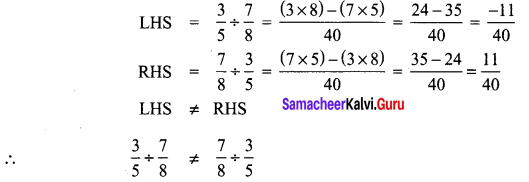∴ Subtraction of rational numbers is not commutative

Question 3.
Is $$\frac{3}{5} \div \frac{7}{8}=\frac{7}{8} \div \frac{5}{3}$$? So, what do you conclude?
Solution: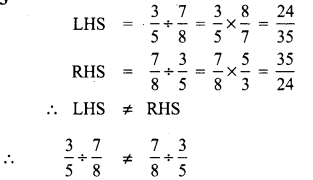∴ Commutative property not hold good for division of rational numbers.

Try This (Textbook Page No. 26)

Question 1.
Check whether the associative property holds for subtraction and division.
Solution:
Consider the rational numbers $$\frac{2}{3}$$, $$\frac{1}{2}$$ and $$\frac{3}{4}$$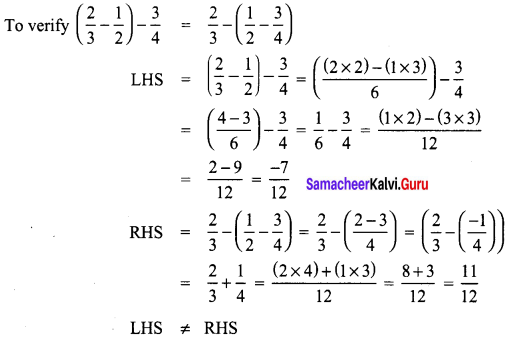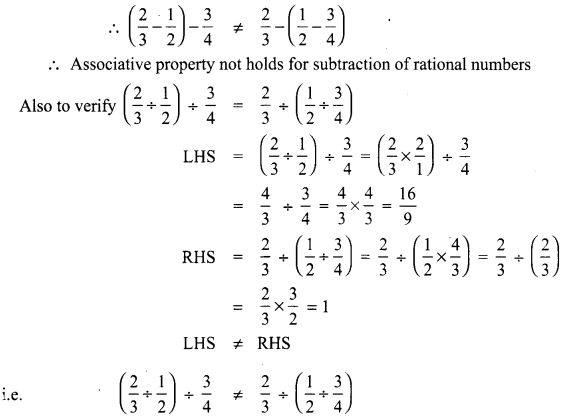∴ Associative property does not hold for division of rational numbersQuestion 2.
Observe that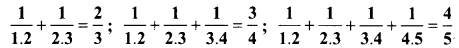Use your reasoning skills, to find the sum of the first 7 numbers in the pattern given above.
Solution: